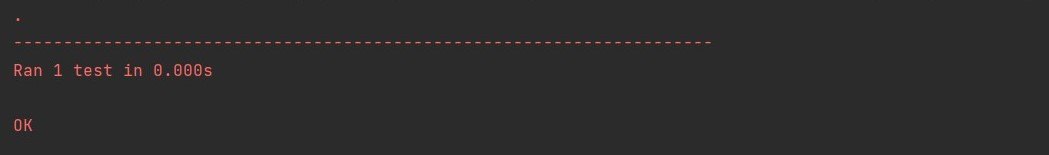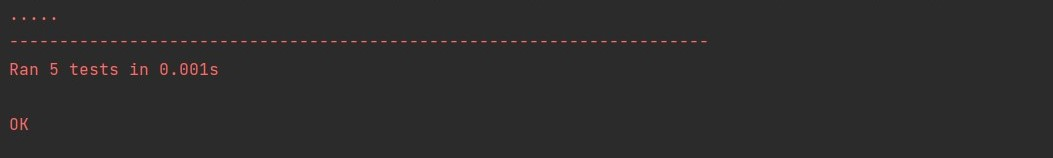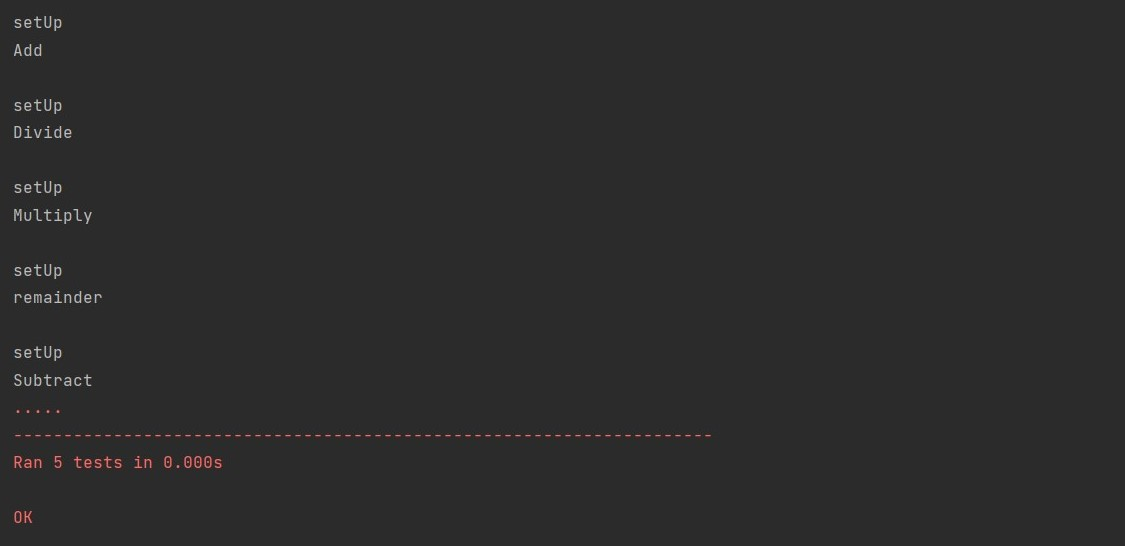# Python Unittest Setup

Migel Hewage Nimesha Sep 22, 2022

Unit testing checks whether a particular code unit or a module performs as the developer expected. Most of the time, the unit of code we test is a function.

Likewise, we can test all the functions. As a best practice, at least in the development process, we should perform unit tests.

So, in the early stage of the development process, it detects bugs, and we can fix them without spending much time. If we skip these tests, it will be hard to fix the errors when continuing with the project.

## Python `unittest` Setup

Python provides a different framework for unit testing called `unittest`. The `unittest` framework offers a few features.

The tests we perform using this method are independent of each other. In other words, the success, or the failure of a test, does not depend on other test results.

It also provides `setup()` and `teardown()` functions and test automation. The `setup()` function allows us to set the instructions to execute before each test method.

The `teardown()` function will describe the instructions running after each test method.

`unittest` gives us some essential concepts to use in unit testing, such as test fixture, test case, test suite, and test runner. This article discusses building a `setup()` function for unit testing using the `unittest` framework.

### Create Functions to Test

First, we need to build some functions to test. Let’s create four functions to add, subtract, multiply, divide two numbers, and get the remainder.

``````def add(a, b):
return a + b

def subtract(a, b):
return a - b

def multiply(a, b):
return a * b

def divide(a, b):
return a / b

def remainder(a, b):
return a % b
``````

We can test the above functions using the `print()` function, but it’s not very practical regarding hundreds or thousands of codes. So, let’s create a module to test the functions.

### Create the Test Module

We should name our test module according to the naming convention. We use `test_` as the first part of the name and then the module’s name.

Let’s create our test module for this tutorial as `test_calc.py`.

Naming convention:

``````test_<name>
``````

Then we have to import the `unittest` framework and `numCal` module to our test module. `unittest` includes it in the standard library, so we can directly import it. `numCal` is the module we need to test.

``````import unittest
import numCal
``````

As the next step, we can create a class that inherits from `unittest.TestCase` so we will have access to more testing capabilities within that class.

``````class TestNumCal(unittest.TestCase):
``````

Inside the class, we create methods for each function. We need to name the methods according to the naming convention when naming them.

When we run the test module, it will know which method represents the tests because the first part of the name is the test. Otherwise, it will not identify the test methods and skip them.

First, let’s create a test method for `add()` function.

``````def test_add(self):
``````

As in every method, `self` is the first argument of the `test_add()` method. We’ve used the `assertEqual()` method in the second line.

Since our `TestNumCal` class inherits from `unittest.TestCase`, we can access all the available assert methods. Methods such as `assertEqual()`, `assertNotEqual()`, `assertTrue()`, `assertFalse()` and `assertIn()` can be used in unit testing and there are lot more.

The `assertEqual()` method checks the equality between the value that returns from the function and the value we expect.

Then we need to set the test module to run directly from the editor. Otherwise, it will not run when we try to execute the test module.

So, for that, we can use the below code.

``````if __name__ == '__main__':
unittest.main()
``````

Now our code should look like this.

``````import unittest
import numCal

class TestNumCal(unittest.TestCase):

if __name__ == '__main__':
unittest.main()
``````

Now we can test the `add()` function by running the `test_calc.py` module.

Output:Here, the dot indicates that the test passed. If the test fails, `F` will appear, and if there is an error, an error message will occur.

Below, the dot shows how many units were tested.

Now let’s create four more test methods to test the remaining functions.

``````import unittest
import numCal

class TestNumCal(unittest.TestCase):

def test_subtract(self):
self.assertEqual(numCal.subtract(10, 5), 5)

def test_multiply(self):
self.assertEqual(numCal.multiply(10, 5), 50)

def test_divide(self):
self.assertEqual(numCal.divide(10, 5), 2)

def test_remainder(self):
self.assertEqual(numCal.remainder(10, 5), 0)

if __name__ == '__main__':
unittest.main()
``````

According to each function, we should change the test method name.

Output:The hard part here is the value for each function needs to be set in each test method. It will be a problem when we have hundreds of test methods.

If the values need to be changed, we must review each test method and change them.

We can build the `setup()` function to overcome this. Through this method, we only need to declare the values once. Then before each method, it executes.

### Create `setUp()` Function

``````def setUp(self):
print("\nsetUp")
self.num1 = 10
self.num2 = 5
``````

After setting up the `setup()` function, the arguments for the functions also need to be changed. First, let’s change the `test_add` method.

``````def test_add(self):
``````

As mentioned above, we can make the changes to the remaining methods, and our complete code should look like the one below.

Full code:

``````import unittest
import numCal

class TestNumCal(unittest.TestCase):

def setUp(self):
print("\nsetUp")
self.num1 = 10
self.num2 = 5

def test_subtract(self):
print("Subtract")
self.assertEqual(numCal.subtract(self.num1, self.num2), 5)

def test_multiply(self):
print("Multiply")
self.assertEqual(numCal.multiply(self.num1, self.num2), 50)

def test_divide(self):
print("Divide")
self.assertEqual(numCal.divide(self.num1, self.num2), 2)

def test_remainder(self):
print("remainder")
self.assertEqual(numCal.remainder(self.num1, self.num2), 0)

if __name__ == '__main__':
unittest.main()
``````

Output:In the output, five dots indicate that the tests have passed and the number of tests that ran. Below it, the values have been set before each test; to identify that, we’ve put `print()` functions.

So before the test methods start, the `setup()` function executes the defined instructions, and the tests happen using them.

## Conclusion

In this article, we’ve learned what unit testing and `unittest` framework are and how to build a test module. Most importantly, we learned how to use the `setup()` function to set some defined instructions to execute before each test method.

The `setup()` function comes in handy when there are many tests to run and when we need to change the values for the arguments.

Nimesha is a Full-stack Software Engineer for more than five years, he loves technology, as technology has the power to solve our many problems within just a minute. He have been contributing to various projects over the last 5+ years and working with almost all the so-called 03 tiers(DB, M-Tier, and Client). Recently, he has started working with DevOps technologies such as Azure administration, Kubernetes, Terraform automation, and Bash scripting as well.